#2018 iT 邦幫忙鐵人賽DAY 14
5
AI & Machine Learning

## 循環神經網路(Recurrent Neural Network, RNN)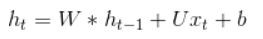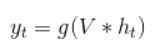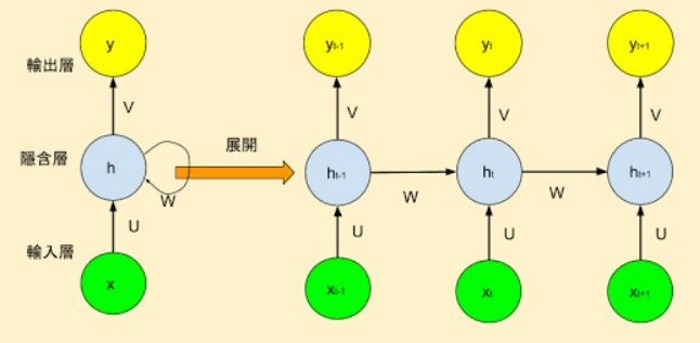• 我不吃辣，所以，我點『蝦仁豆腐』
• 我吃辣 ，所以，我點『麻婆豆腐』

1. 一對一(one to one)：固定長度的輸入(input)及輸出(output)，即一般的 Neural Network 模型。
2. 一對多(one to many)：單一輸入、多個輸出，例如影像標題(Image Captioning)，輸入一個影像，希望偵測影像內多個物體，並一一給予標題，這稱之為『Sequence output』。
3. 多對一(many to one)：多個輸入、單一輸出，例如情緒分析(Sentiment Analysis)，輸入一大段話，判斷這段話是正面或負面的情緒表達，這稱之為『Sequence input』。
4. 多對多(many to many)：多個輸入、多個輸出，例如語言翻譯(Machine Translation)，輸入一段英文句子，翻譯成中文，這稱之為『Sequence input and sequence output 』。
5. 另一種多對多(many to many)：『同步』(Synchronize)的多個輸入、多個輸出，例如視訊分類(Video Classification)，輸入一段影片，希望為每一幀(Frame)產生一個標題，這稱之為『Synced sequence input and output』。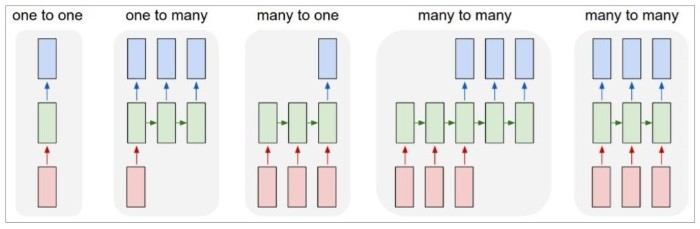## 實作

``````# 導入函式庫
import numpy as np
from keras.datasets import mnist
from keras.utils import np_utils
from keras.models import Sequential
from keras.layers import SimpleRNN, Activation, Dense
from keras.optimizers import Adam

# 固定亂數種子，使每次執行產生的亂數都一樣
np.random.seed(1337)

# 載入 MNIST 資料庫的訓練資料，並自動分為『訓練組』及『測試組』
(X_train, y_train), (X_test, y_test) = mnist.load_data()

# 將 training 的 input 資料轉為3維，並 normalize 把顏色控制在 0 ~ 1 之間
X_train = X_train.reshape(-1, 28, 28) / 255.
X_test = X_test.reshape(-1, 28, 28) / 255.
y_train = np_utils.to_categorical(y_train, num_classes=10)
y_test = np_utils.to_categorical(y_test, num_classes=10)

# 建立簡單的線性執行的模型
model = Sequential()
# 加 RNN 隱藏層(hidden layer)
model.add(SimpleRNN(
# 如果後端使用tensorflow，batch_input_shape 的 batch_size 需設為 None.
# 否則執行 model.evaluate() 會有錯誤產生.
batch_input_shape=(None, 28, 28),
units= 50,
unroll=True,
))
# 加 output 層
model.add(Dense(units=10, kernel_initializer='normal', activation='softmax'))

# 編譯: 選擇損失函數、優化方法及成效衡量方式
model.compile(loss='categorical_crossentropy', optimizer='adam', metrics=['accuracy'])

# 一批訓練多少張圖片
BATCH_SIZE = 50
BATCH_INDEX = 0
# 訓練模型 4001 次
for step in range(1, 4001):
# data shape = (batch_num, steps, inputs/outputs)
X_batch = X_train[BATCH_INDEX: BATCH_INDEX+BATCH_SIZE, :, :]
Y_batch = y_train[BATCH_INDEX: BATCH_INDEX+BATCH_SIZE, :]
# 逐批訓練
loss = model.train_on_batch(X_batch, Y_batch)
BATCH_INDEX += BATCH_SIZE
BATCH_INDEX = 0 if BATCH_INDEX >= X_train.shape else BATCH_INDEX

# 每 500 批，顯示測試的準確率
if step % 500 == 0:
# 模型評估
loss, accuracy = model.evaluate(X_test, y_test, batch_size=y_test.shape,
verbose=False)
print("test loss: {}  test accuracy: {}".format(loss,accuracy))

# 預測(prediction)
X = X_test[0:10,:]
predictions = model.predict_classes(X)
# get prediction result
print(predictions)

# 模型結構存檔
from keras.models import model_from_json
json_string = model.to_json()
with open("SimpleRNN.config", "w") as text_file:
text_file.write(json_string)

# 模型訓練結果存檔
model.save_weights("SimpleRNN.weight")
``````

python RNN.py

## 程式說明

1. 如果使用 Sequential 模型，且設定 batch_input_shape 維度，表示當批的output作為下一批的樣本初始狀態( initial states for the samples in the next batch)，稱之為『stateful』。
2. 如果要在後續的隱藏層要重置狀態(state)，可呼叫.reset_states()。
3. RNN 會考慮同一層前面的輸出，當參數 unroll=True 時，表示計算時會先展開結構，如下圖一，它會使用較多的記憶體，但會縮短計算時間，反之 unroll=False，如下圖二。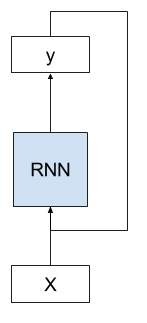model.add(Bidirectional(SimpleRNN(10)))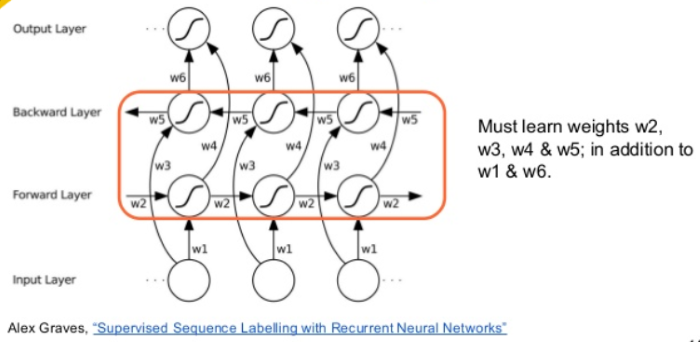### 1 則留言

0